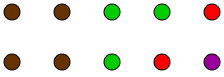### Home > MC1 > Chapter 9 > Lesson 9.2.3 > Problem9-81

9-81.

A bag contains $4$ brown marbles, $3$ green marbles, $2$ red marbles, and $1$ purple marble. Calculate the theoretical probability of drawing each color, and write each answer as a fraction, as a percent, and as a decimal.

The marbles are shown below to help you visualize. There are $10$ marbles total.The probability of drawing the purple marble is $10\%$, $0.1$, or one-tenth.